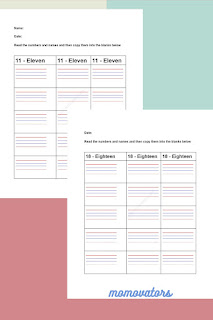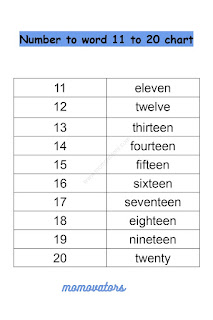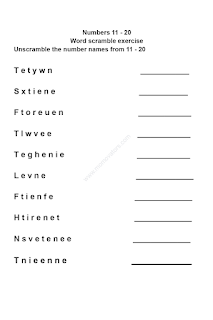-->

# Number names 11 to 20 worksheets

## 10+ number names 11 to 20 worksheets

Are you looking for the number names 11 20 worksheet PDF? Here, you will find 10+ exercises to work on the number words 11 to 20. Build a strong foundation of the number names concept with our worksheets. You can find the download link for these 11 20 names worksheets at the bottom of this post.

As you all know, math helps to develop problem-solving, critical thinking, and analytical thinking skills. So, create a fun and successful learning environment with our Momovators worksheets. In this post, you will find more than 10 exercises on number names from 11 to 20 in English.

### Insights of Number to words from 11 to 20 PDF:number words 11 20 PDF

Here, you will find 10+ activities that might help your kids or students to practice number names.

#### 1. 11 to 20 number names charts

To begin with, you will find the number names chart of 11 to 20.11 20 number words chart

Number to word chat from 11 to 20 in English are:

11 - eleven
12 - twelve
13 - thirteen
14 - fourteen
15 - fifteen
16 - sixteen
17 - seventeen
18 - eighteen
19 - nineteen
20 - twenty

#### 2. Eleven to Twenty Number names matching activity

In this exercise, the child needs to map the numbers with their corresponding number names. This activity makes the learning of number names easy.

#### 3. 11 20 Number Names Practice worksheet

You can use this worksheet to practice the number names. There are two empty columns for practicing the number words.

#### 4. Unscrabling Number to words worksheet

The number names are in a scrambled manner. The child needs to unscramble the number names. This activity helps to enhance number word recognition and vocabulary skills.unscramble 11 20 PDF

#### 5. Arrange the number names in a sequence

The child needs to arrange the number names in an ascending order or descending order.

#### 6. Circle the number names worksheet

This worksheet would be fun for the children. The children need to circle the right number names to the respective number. You can use this worksheet in the initial phase. So that, the child can recognize the number name before learning the spellings.

Look at each number and circle the correct number name.

A. 16

a.eighteen

b sixteen

c. nine

B. 12

a. thirteen

b. fourteen

c. twelve

C. 20

a. fifteen

b. twenty

c. twelve

D. 11

a. eleven

b. twelve

c. thirteen

E. 18

a. sixteen

b. twenty

c. eighteen

### Conclusion